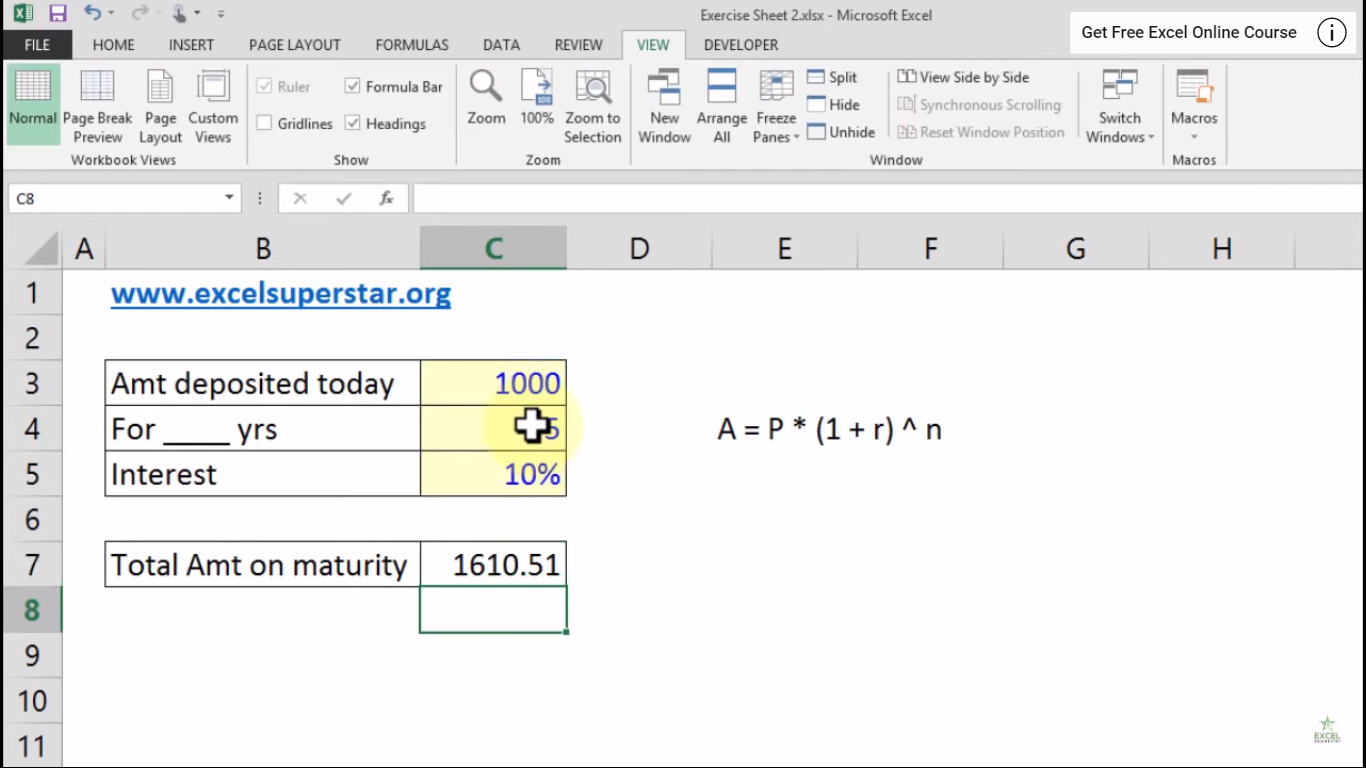# Excel Calculating Fixed Deposit maturity amount Part – 2In this blog, we will learn about Calculating Fixed Deposit maturity amount in Excel

Example: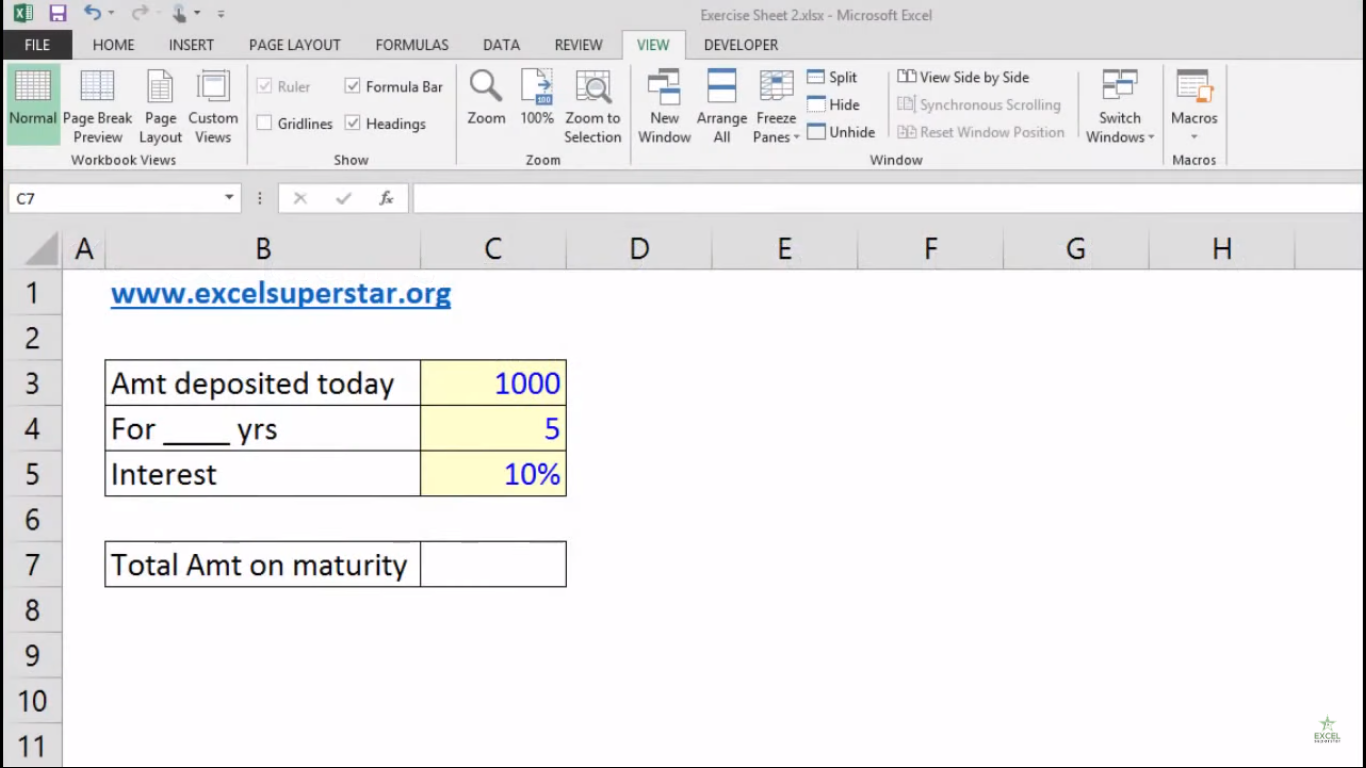If you have RS 1000 invested in Fixed Deposit where the bank’s Annual Interest Rate is 10%. Then how much total amount you will be getting after 5 years?
Here we will be applying the Simple Interest formula to calculate the total amount

A=P*(1+r) ^ n

A – Amount at the end of your maturity period

P – Principal

* – Multiply Sign which you will get on your digit 8 keyboard

r – R means rate of interest

^ – To the power sign which you will get on your digit 6 keyboard

n – Number of years

1. Write = and select the Principal Amount =C3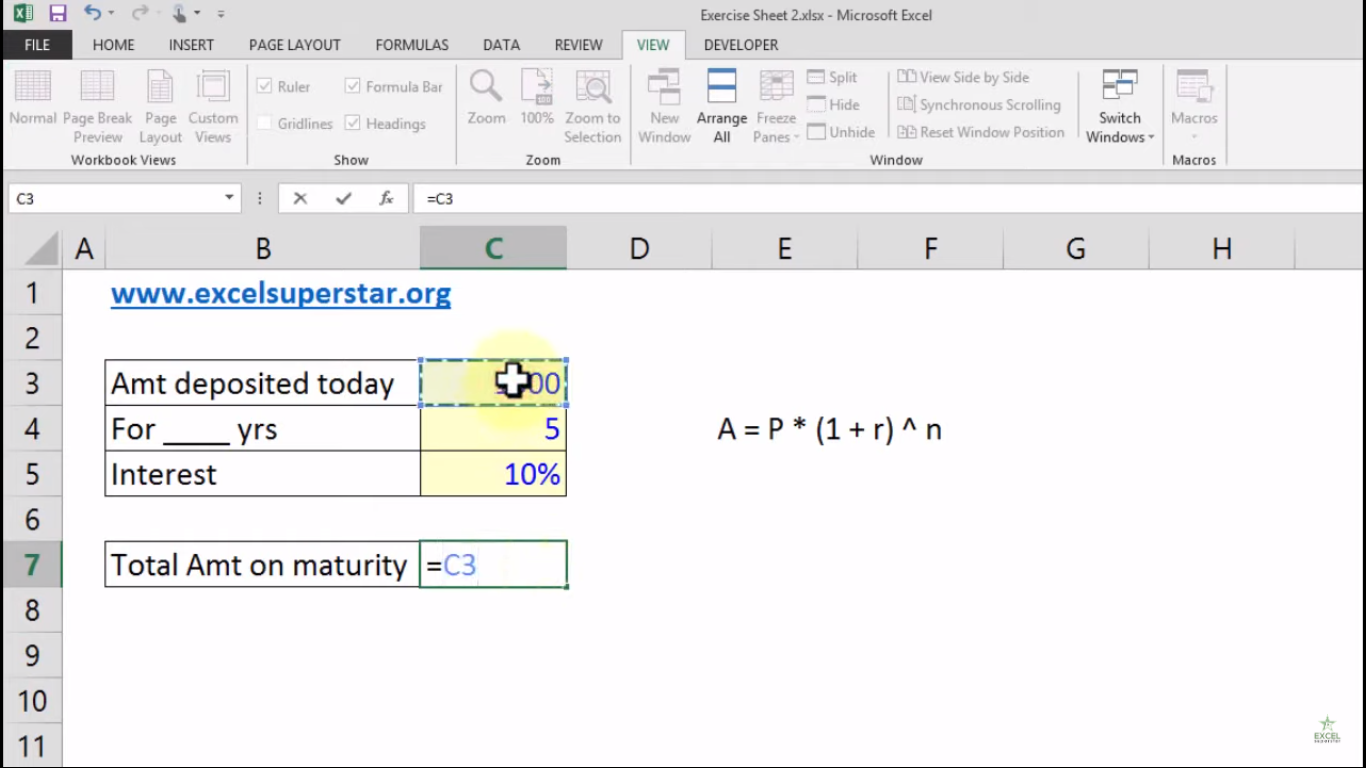2. Apply the Multiply Sign *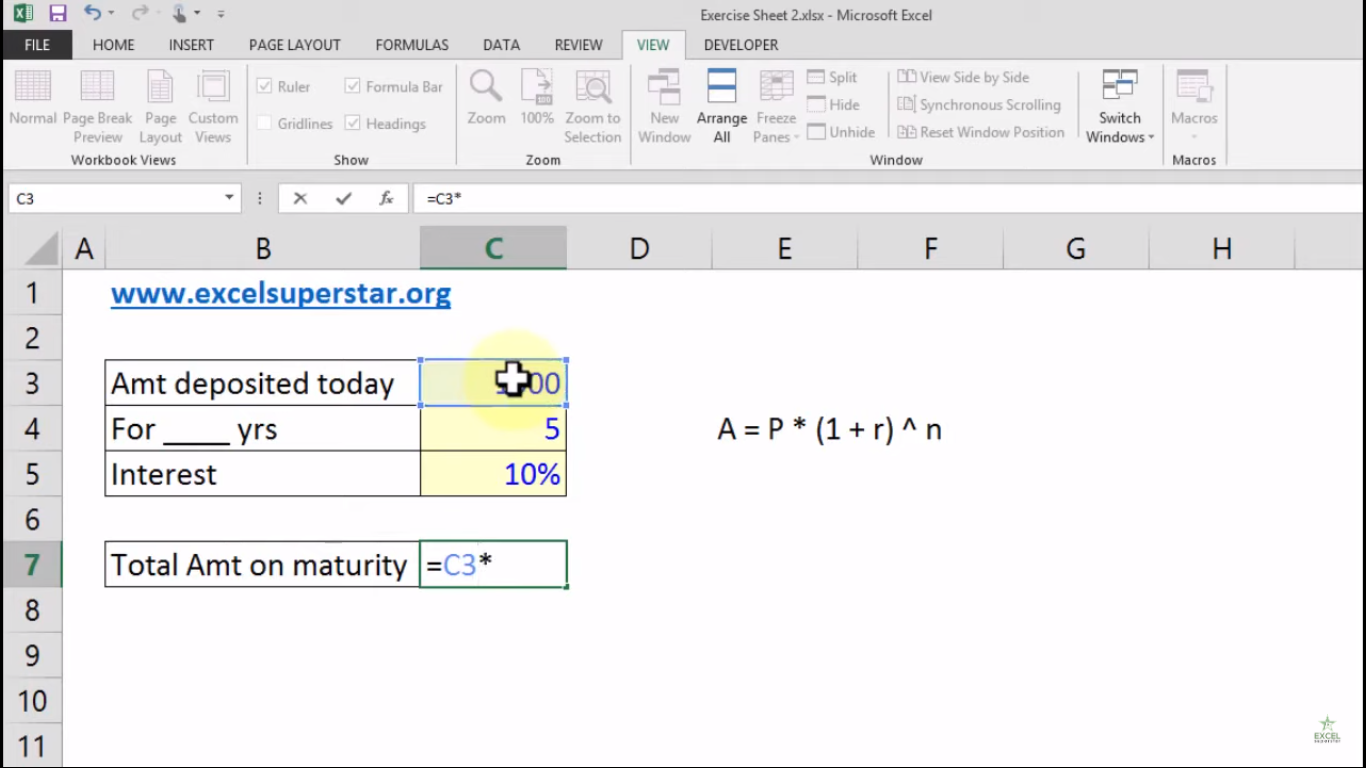Note – Multiply Sign you will get on your digit 8 keyboard

3. Open the bracket and write 1+ and select the Interest and close the bracket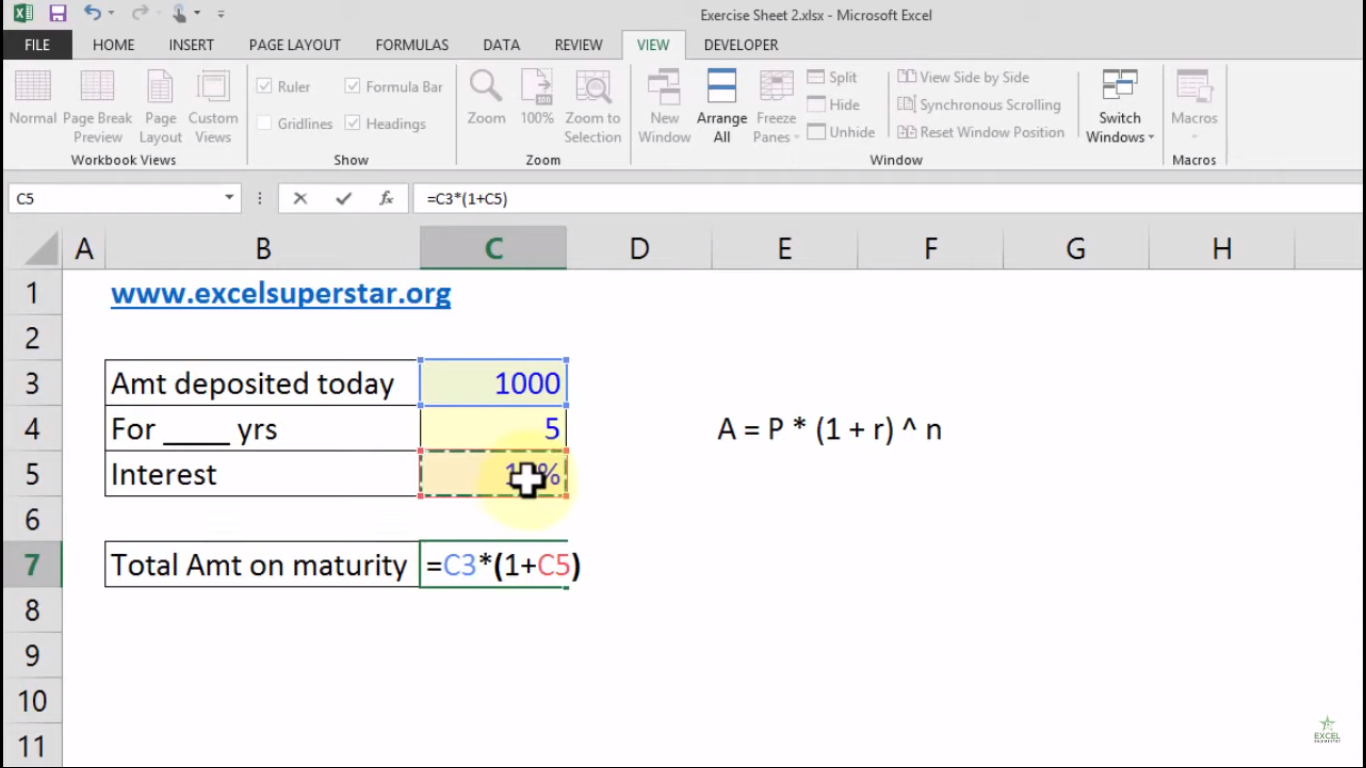4. Apply to the power sign ^ and choose the number of years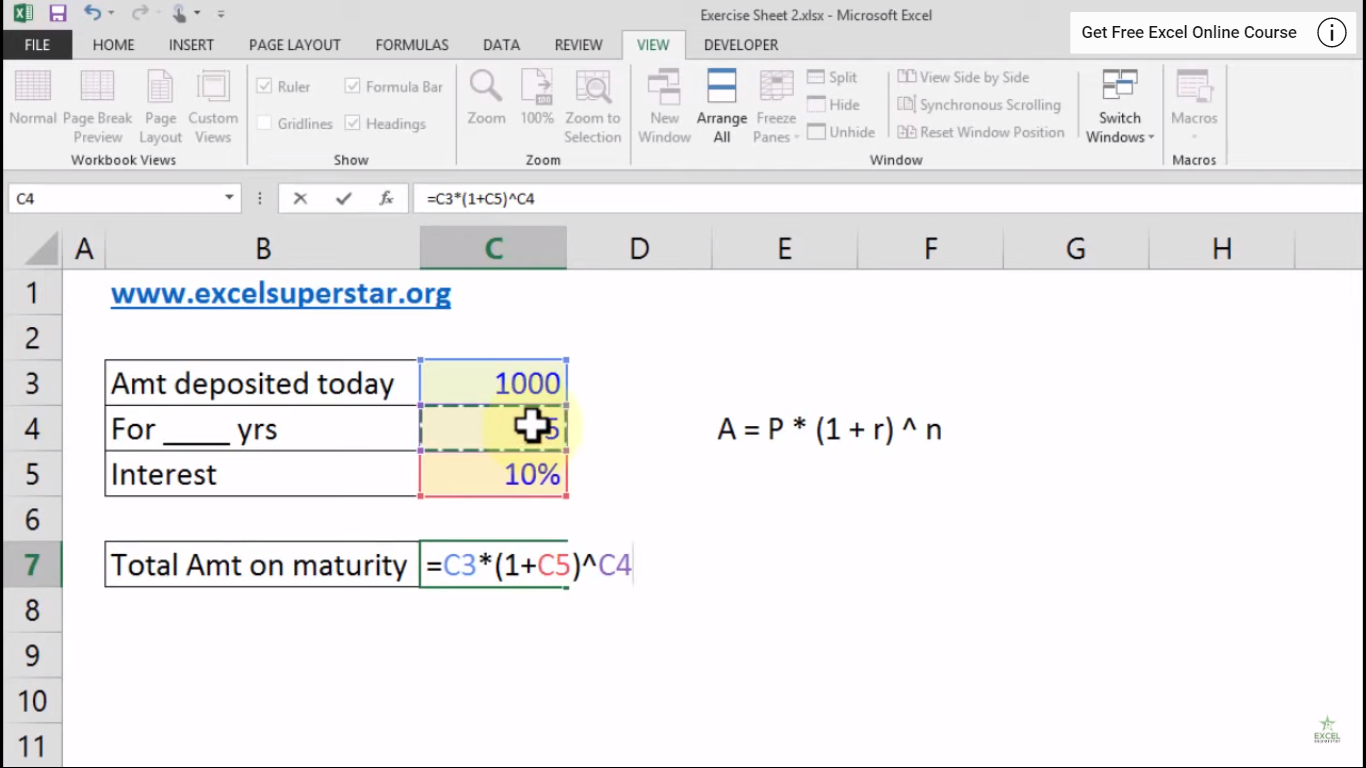5. Press Enter